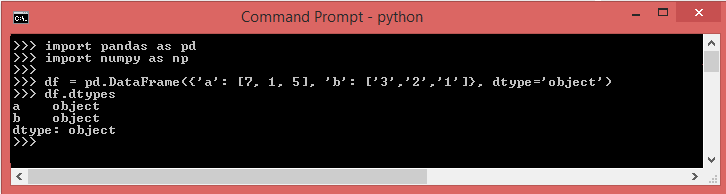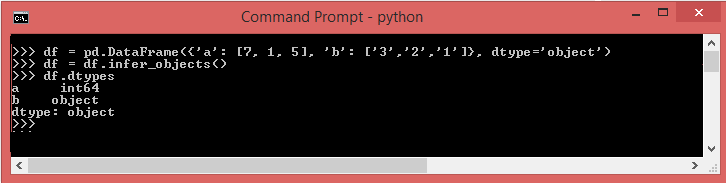DS Tutorial

# Pandas DataFrame operations

Data types are essential for programming languages to understand how to store, manipulate, and interpret data. In Python, data types play a crucial role in determining the format of individual rows and columns in datasets. The Pandas DataFrame is a powerful 2-dimensional data structure that allows data to be organized into rows and columns with corresponding labels, making it efficient for data analysis and manipulation.

1. Types of Data
2. Numeric Data Types
3. Text Data Type

The numeric data types include integers (int) and floating-point numbers (float). Text data, also known as strings, are represented as objects in Pandas or strings in native Python. Pandas may use slightly different names for data types compared to native Python, but they essentially serve the same purpose. For example, Pandas represents integers with 'int64' and floating-point numbers with 'float64'.

## How to Check the Data Type in Pandas DataFrame

Checking data types in a DataFrame serves two essential purposes. Firstly, Pandas automatically assigns data types based on the encoding it detects in the original dataset. However, this assignment may not always be accurate, and verifying the data types allows you to ensure correctness and consistency in your analysis.

Secondly, the data type of a column in a Pandas DataFrame or Series is referred to as dtype. You can use the dtype property to examine the data type of a specific column, which is helpful for understanding the structure of the data and making informed decisions during data processing and analysis.

You can use the following syntax to check the data type of all columns in Pandas DataFrame :

## df.dtypes

Alternatively, you may use the syntax below to check the data type of a specific column in a DataFrame:

df['DataFrame Column'].dtypes

## How to change column type in pandas?

You have four main options for converting types in pandas:

1. astype()
2. to_numeric()
3. infer_objects()
4. convert_dtypes()

## astype()

The astype() method in Pandas is primarily used for converting a Pandas object (e.g., DataFrame, Series) to a specified data type. It allows you to change the data type of the entire DataFrame or Series to a new specified type.

Additionally, the astype() method can also be used to convert an existing column in the DataFrame to a categorical data type. This can be particularly useful for memory optimization and improving performance when dealing with categorical data.

example
df = df.astype(int) # convert all columns to int64
df = df.astype({"x": int, "y": complex}) # column "x" to int64 dtype and "y" to complex type
s = s.astype(np.float16) # Series to float16 type
s = s.astype(str) # Series to Python strings
s = s.astype('category') # Series to categorical type

## to_numeric()

The to_numeric() method in Pandas is used to convert a Series or DataFrame column to numeric data type, trying to change strings or non-numeric objects into integers or floating-point numbers as appropriate.

When you have a column that contains both numeric and non-numeric data, Pandas will attempt to convert the non-numeric elements into their appropriate numeric representation. If it encounters any invalid or unconvertible data, it will raise an error or return NaN (Not a Number) for those elements.

This method is helpful when you need to clean and transform data, especially when dealing with messy datasets where some values may be improperly formatted or have mixed data types.

df["a"] = pd.to_numeric(df["a"]) # column "a" of a DataFrame
df[["a", "b"]] = df[["a", "b"]].apply(pd.to_numeric) # convert columns "a" and "b"

## infer_objects()

The infer_objects() method in Pandas is used to automatically infer and convert columns of a DataFrame that have an object datatype to a more specific type. This method is particularly useful when you have DataFrame columns with mixed data types or columns that were imported as generic objects, and you want to convert them to their appropriate types for more efficient storage and computation.

The infer_objects() method analyzes the data in each column and attempts to infer the best data type for that column based on the actual values present in the column. It will convert columns to more specific data types like integers, floats, or other specialized types like datetime, category, etc., whenever possible.Using infer_objects() , you can change the type of column 'a' to int64:## convert_dtypes()

The convert_dtypes() method in Pandas is a powerful tool for automatically converting the default assigned data types to the most suitable data types based on the actual values present in each column. It was introduced in Pandas version 1.0.0 and provides several advantages over other methods like astype() or infer_objects().

One of the significant advantages of using convert_dtypes() is its support for the new missing value representation pd.NA, which was introduced in Pandas to represent missing values in a more intuitive and consistent way, especially for non-numeric data types. Prior to this, Pandas used NaN for missing values, which was primarily used for numeric data types.

Using convert_dtypes(), you can automatically convert columns with missing values to the appropriate data type, including the usage of pd.NA, which enhances the consistency and expressiveness of missing value handling in non-numeric columns.

Moreover, convert_dtypes() also provides better handling of categoricals and other specialized data types, making it a more convenient and comprehensive method for automatically converting data types in a Pandas DataFrame.

import pandas as pd import numpy as np # creating a dataframe df = pd.DataFrame({"Roll_No.": ([101, 102, 103]), "Name": ["John", "Doe", "Bill"], "Result": ["Pass", "Fail", np.nan], "Promoted": [True, False, np.nan], "Marks": [80.34, 36.6, np.nan]}) # printing the dataframe print("PRINTING DATAFRAME") display(df) # checking datatype print() print("PRINTING DATATYPE") print(df.dtypes) # converting datatype print() print("AFTER CONVERTING DATATYPE") print(df.convert_dtypes().dtypes)

### Conclusion

Pandas DataFrame operations allow for efficient and flexible data manipulation in Python. With methods like astype(), to_numeric(), infer_objects(), and convert_dtypes(), you can easily convert and handle data types, making data analysis and cleaning tasks more straightforward and concise. Additionally, these operations enable seamless handling of missing values, support for categoricals, and provide improved performance when working with large datasets.